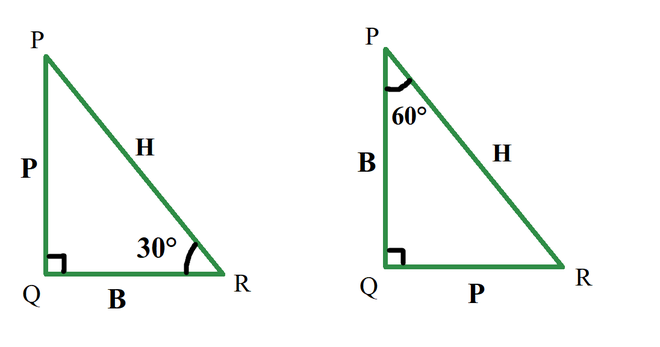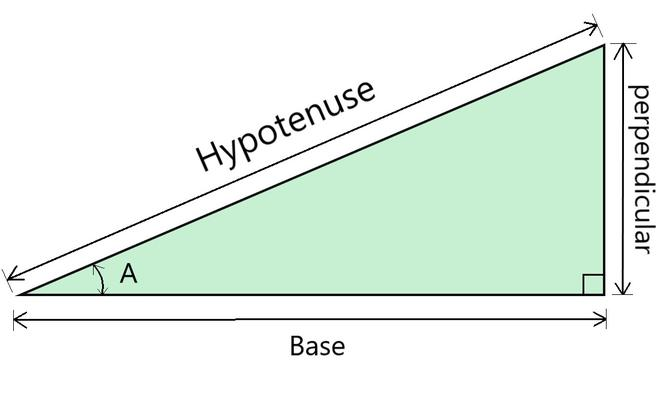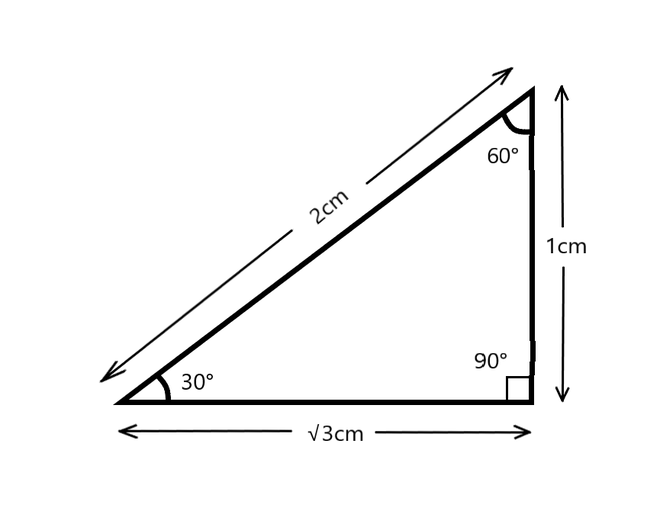Open in App
Not now

# How are sine, cosine, and tangent calculated?

• Difficulty Level : Medium
• Last Updated : 06 Oct, 2021

Trigonometry is the branch of mathematics that deals with the relationship of sides with angles in a triangle. With trigonometry finding out the heights of big mountains or towers is possible, also in astronomy, it is used to find the distance between stars or planets and is widely used in physics, architecture, and GPS navigation systems. Trigonometry is based on the principle that “If two triangles have the same set of angles then their sides are in the same ratio”. Side length can be different but side ratios are the same.

### Right angle triangle

Trigonometric ratios are defined only for a right-angle triangle. In a right-angled triangle, there is a 90° angle and the other two angles are less than 90°, concerning these angles each side is given a name as perpendicular base and hypotenuse. Let’s see what the Perpendicular, base, and hypotenuse of a right-angled triangle are,

• Hypotenuse: It is a side opposite to 90°. it is the largest side.
• Perpendicular: The side in front of the angle or opposite to an angle is perpendicular.
• Base: A base is one of the sides which touches angle,

Note Hypotenuse can never be considered as base or perpendicular.

In a right angle triangle, for angle other than 90° is formed by two sides of which one is the hypotenuse. the other side which contains or touches the angle is the base and the side which do not touches the angle is perpendicular.As shown in the above diagram for the same triangle if considered angle 30° the perpendicular is the side PQ, but if considered angle 60° the perpendicular is side QR.

### Trigonometric functions

Trigonometric functions are also called circular functions or trigonometric ratios. are the ratios of the side of a right-angle triangle, They show the relationship between angle and sides, and they are the base of trigonometry. There are six trigonometric functions Sine, Cosine, Tangent, Cosecant, Secant, Cotangent. The sides representations for the six ratios are,

• sin A = Perpendicular / Hypotenuse
• cos A = Base / Hypotenuse
• tan A = Perpendicular / Base
• cot A = Base / Perpendicular
• sec A = Hypotenuse / Base
• cosec A = Hypotenuse / Perpendicular.

### How are sine, cosine, and tangent calculated?

Sine, Cosine, and Tangent also called sin, cos and tan respectively are the most commonly used trigonometric ratios, the other 3 are the reciprocal of these.•  Sin – The sin of an angle A is the ratio of lengths of the perpendicular to the hypotenuse.

sin A = Perpendicular / Hypotenuse

• Cos – The cos of an angle A is the ratio of lengths of the base to the hypotenuse.

cos A = Base / Hypotenuse

• Tan – The tan of an angle A is the ratio of lengths of perpendicular to the base.

tan A = Perpendicular / Base

To calculate these ratios find the length of the sides of the triangle and then take ratios respectively. In order to find length if one of the sides and angle is known, one can easily find the rest of the sides through sine, cos and tan angle. Below is the trigonometric angle values of some important angles.

### Sample problems

Question 1: Consider the following triangle and answer the question below?Find the value of sin, cos, and tan for angle 30°

Solution:

For angle 30°

perpendicular = 1cm, base = √3cm, hypotenuse = 2cm.

Sin(30°) = (p/h)  =  1/2.

Cos(30°) = (b/h) = √3/2.

tan(30°) = (p/b) = 1/2.

Question 2. For the same figure in question 1, find the value of sin, cos, and tan for angle 60°

Solution:

For angle 60°

perpendicular = √3cm, base = 1cm, hypotenuse = 2cm.

Sin(60°) = (p/h)  =  √3/2.

Cos(60°) = (b/h) = 1/2.

tan(60°) = (p/b) = √3/1.

Question 3: In a right-angled triangle, the base to the angle 30° is 18m. Find the length of the Hypotenuse.

Solution:

Given: Base = 18m

Cos = √3/2

B/H = √3/2

18/H = √3/2

H = (18 × 2) / √3

H = 12√3m

Question 4: In a right-angled triangle, the side opposite to the angle 30° is 14m. Find the length of the Hypotenuse.

Solution:

Given: Perpendicular = 14m

Sin 30 = 1/2

P/H = 1/2

14/H = 1/2

H = 14 × 2

H = 28m.

My Personal Notes arrow_drop_up